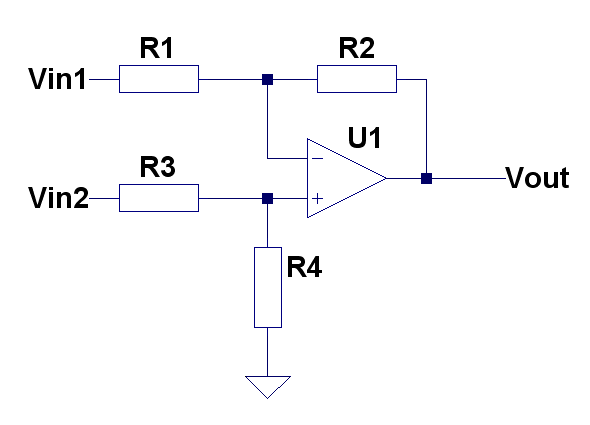# Difference amplifierVout=V2(R1+R2)/(R3+R4)*(R4/R1)+V1*(R2/R1)

For R1=R3 and R2=R4

Vout =(V2-V1)(R2/R1)

R1||R2 = R3||R4  – for minimum offset error due input bias current